# PolynomialsPage 1

#### WATCH ALL SLIDES

Slide 1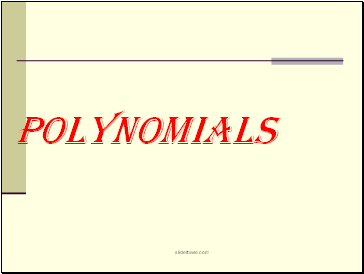POLYNOMIALS

Slide 2## Polynomials

A polynomial is a function of the form

where the

are real numbers and n is a nonnegative integer.

The domain of a polynomial function is the set of real numbers

Slide 3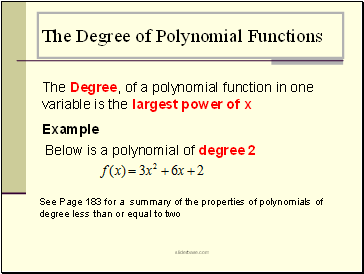## The Degree of Polynomial Functions

The Degree, of a polynomial function in one variable is the largest power of x

Example

See Page 183 for a summary of the properties of polynomials of degree less than or equal to two

Below is a polynomial of degree 2

Slide 4## Properties of Polynomial Functions

The graph of a polynomial function is a smooth and continuous curve

A smooth curve is one that contains no Sharp corners or cusps

A polynomial function is continuous if its graph has no breaks, gaps or holes

Slide 5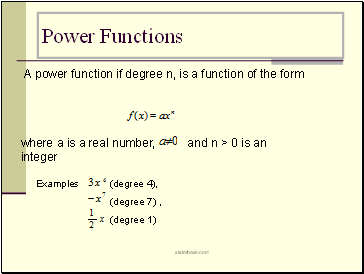## Power Functions

A power function if degree n, is a function of the form

where a is a real number, and n > 0 is an integer

Examples (degree 4),

(degree 7) ,

(degree 1)

Slide 6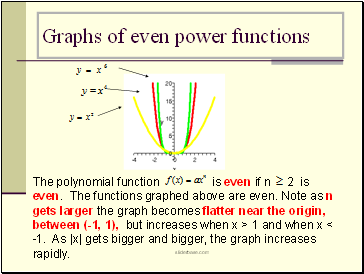## Graphs of even power functions

The polynomial function is even if n 2 is even. The functions graphed above are even. Note as n gets larger the graph becomes flatter near the origin, between (-1, 1), but increases when x > 1 and when x < -1. As |x| gets bigger and bigger, the graph increases rapidly.

Slide 7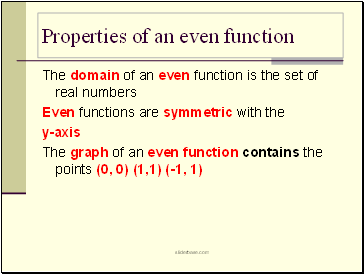## Properties of an even function

The domain of an even function is the set of real numbers

Even functions are symmetric with the

y-axis

The graph of an even function contains the points (0, 0) (1,1) (-1, 1)

Slide 8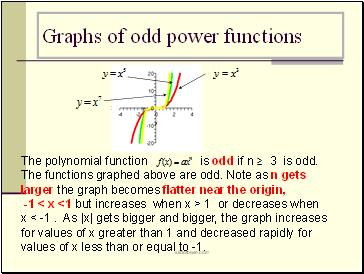## Graphs of odd power functions

The polynomial function is odd if n 3 is odd. The functions graphed above are odd. Note as n gets larger the graph becomes flatter near the origin,

-1 < x <1 but increases when x > 1 or decreases when

x < -1 . As |x| gets bigger and bigger, the graph increases for values of x greater than 1 and decreased rapidly for values of x less than or equal to -1.

Slide 9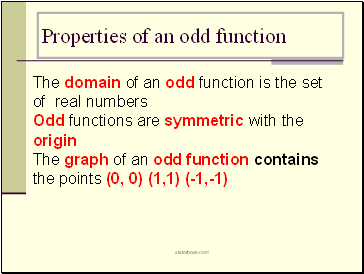## Properties of an odd function

The domain of an odd function is the set of real numbers

Odd functions are symmetric with the

origin

The graph of an odd function contains the points (0, 0) (1,1) (-1,-1)

Go to page:
1  2